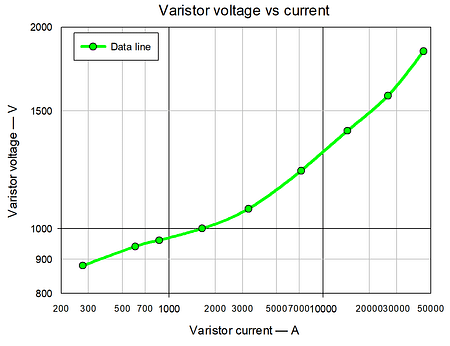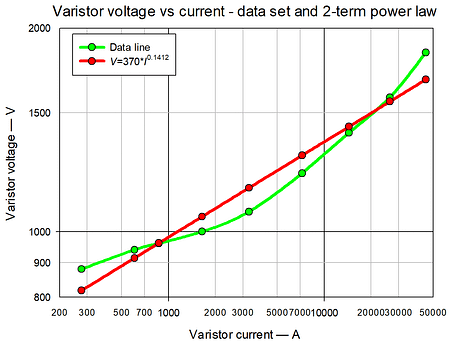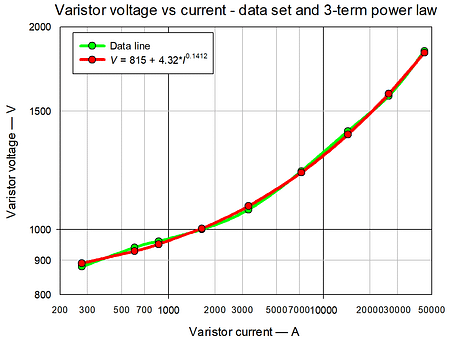# Modelling an MOV voltage versus current characteristic

The previous “There’s an R in varistor” post showed how the varistor is really a current controlled component. In modelling the varistor resistance or voltage, it is logical that current should be the controlling parameter used in the data set curve fitting equations. The IEC 61051-1 ED3 includes a 2-term power law equation, V = BxI, in its varistor definition. However, this is a misdirection as it will be shown this equation is only valid over a small range of currents.

This post uses the same data set for curve fitting as the previous “There’s an R in varistor” post, see the Figure below:Using the IEC 61051-1, ED3 equation the best fit requires these values,  V = 370xI0.1412 , the result is shown in the Figure below:Clearly, although the equation values minimize errors, the equation is not a good fit to the data. Over a small current range, say 10:1, the equation is useful. Here the current range is 160:1, which is too large for the 2-term power law equation. By changing to a 3-term power law equation, V = A + BxIc, a much better fit can be achieved as shown in the Figure below.In this case the best fit equation was V = 815 + 4.32xI0.1412. The failing of this equation is that the voltage can never be less than 815 V for currents of less than 1 A.

What did the Chinese recommend? For voltage, the equation given was VI*10(3.2306-1.2465*LOG(I)+0.05434*LOG(I)*LOG(I)), which is shown plotted in the Figure below:Although not quite as good a fit as the 3-term power law, the Chinese equation misbehaves at lower currents for example at currents of 50 A, 10 A, 1 A and 0.1 A it returns unrealistic voltages of 930 V, 1093 V, 1700 V and 3400 V. It is always advisable to check what happens when values are used outside the curve fitting data set range.

In summary the IEC 61051-1, ED3, equation needs warning for the appropriate (small) current range it can be used on. Similarly the Chinese equation needs a warning that it returns unrealistic voltage values at lower currents. The 3-term power law is the best of all, but needs the warning that the voltage cannot be lower than 815 V at lower currents.